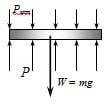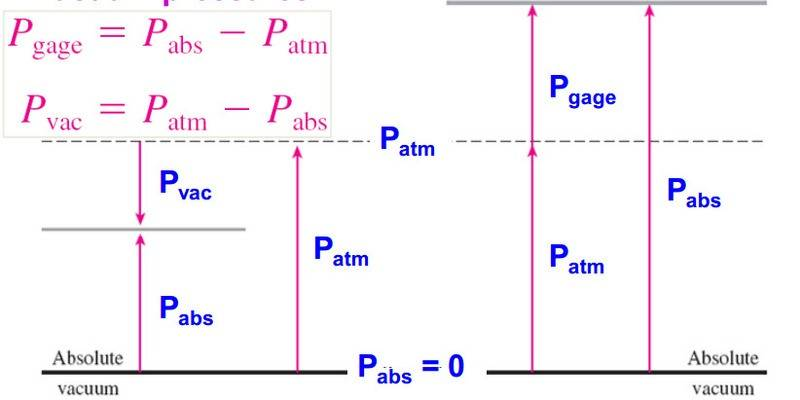# Thermodynamics - Pressure Question

[SOLVED] Thermodynamics - Pressure Question

## Homework Statement

A pressure cooker cooks a lot faster than an ordinary pan by maintaining a higher pressure and temperature inside. The lid of a pressure cooker is well sealed, and steam can escape only through and opening in the middle of the lid. A seperate metal piece , the petcock, sits on top of this opening and prevents steam from escaping untill the pressure force overcomes the weight of the petcock. The periodic escape of the steam in this manner prevents any potentially dangerous pressure buildup and keeps the pressure inside at a constant value. Determine the mass of the petcock of a pressure cooker whose operations pressure is 100 KPa gage and has an opening cross-sectional area of 4 mm^2 . assume an atomspheric pressure of 101 KPa, and a draw of the petcock

My Question:

I already have the solution to this question and it is stated that:

Atmospheric pressure is acting on all surfaces of the petcock, which balances itself out. Therefore, it can be disregarded in calculations if we use the gage pressure as the cooker pressure. A force balance on the petcock (ƩFy = 0) yields

Here is the diagram given:If the atmpospheric pressure is still pushing down on the petcock, how can we just ignore it?

Last edited:

Andrew Mason
Homework Helper
If the atmpospheric pressure is still pushing down on the petcock, how can we just ignore it?
You don't ignore it. You just do not have to take it into account explicitly if you use the gage pressure in the pot. The gage pressure takes it into account for you.

The actual pressure in the pot is Pactual = Pgage + Patm.

AM

You don't ignore it. You just do not have to take it into account explicitly if you use the gage pressure in the pot. The gage pressure takes it into account for you.

The actual pressure in the pot is Pactual = Pgage + Patm.

AM

So basically whenever the term guage pressure is used, it gives you the difference between the internal and atmospheric pressure?

I thought that was P$_{vacuum}$? My notes shows a diagram of P$_{vacuum}$ and P$_{guage}$. The P$_{vacuum}$ one shows like it gives you the resultant pressure between the atmosphere and the internal pressure (image below) which looks more like what we have in the diagram in my first post.I apologize if I'm not making any sense but I'm still very lost even though I understand what you're saying. ):

Redbelly98
Staff Emeritus
Homework Helper
So basically whenever the term guage pressure is used, it gives you the difference between the internal and atmospheric pressure?

I thought that was P$_{vacuum}$?
$$P_{gauge} = P_{abs}-P_{atm}$$
$$P_{vac} = P_{atm}-P_{abs}$$
$$P_{gauge} = P_{abs}-P_{atm}$$
$$P_{vac} = P_{atm}-P_{abs}$$